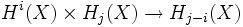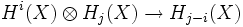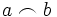# Cap product

## Definition

Let$X$ be a topological space and$R$ a commutative ring. For$i,j$ integers, the cap product is a bilinear map:$H^i(X) \times H_j(X) \to H_{j-i}(X)$

Equivalently it is a linear map:$H^i(X) \otimes H_j(X) \to H_{j-i}(X)$

The cap product turns the direct sum of homology groups into a graded module over the cohomology ring, when viewed as a graded$R$-algebra.

The cap product of$a$ and$b$ is denoted as:$a \frown b$